# B. Dembin: The time constant for Bernoulli percolation is Lipschitz continuous strictly above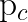Abstract: We consider the standard model of i.i.d. first passage percolation on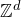given a distribution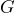on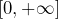(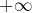is allowed). When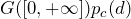. In this case, the travel time between two points is equal to the length of the shortest path between the two points in a bond percolation of parameter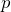. We show that the function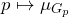is Lipschitz continuous on every interval, where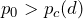.

This is a joint work with Raphaël Cerf.

## Video